Latest Banking jobs   »   Quantitative Aptitude Quiz For IBPS RRB...

# Quantitative Aptitude Quiz For IBPS RRB PO, Clerk Prelims 2021- 26th May

.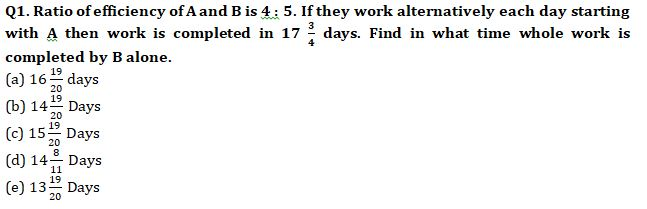Q2. Vessel A contains mixture of milk, water and wine in the ratio 2 : 3 : 5 and vessel B contains mixture of milk and wine in the ratio 3 : 5. If quantity of milk in vessel A is equal to quantity of milk in vessel B and sum of water in A and wine in B is 95L then find the total quantity of mixture in vessel A.
(a) 75L
(b) 100 L
(c) 125 L
(d) 135 L
(e) 150 L

Q3. An amount of (P + 3000) is invested on C.I. at the rate (R + 2)% for two years. If total interest obtained on principal is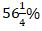then find the value of R.
(a) 25%
(b) 23%
(c) 28%
(d) 30%
(e) 18%

Q4. A man goes 28 km upstream in 7 hours. If he goes the same distance by train then he takes 2 hours.If ratio of speed of boat in still water to speed of train is 3 : 7 then find the time in which boat will cover 40 km in downstream.
(a) 3.5 h
(b) 4 h
(c) 6 h
(d) 4.5 h
(e) 5 h

Q5. Area of a rectangle is ‘r²’ where r is the radius of circle with circumference equal to 88 cm. If length of rectangle is 100% more than the radius of circle then find the breadth of rectangle.
(a) 8 cm
(b) 9 cm
(c) 7 cm
(d) 14 cm
(e) 10 cm

Q6. Abhishek lent Satish Rs.12000 on C.I. at the rate of 20% per annum and at the end of first year Satish borrowed Rs.x more from Abhishek on C.I. at the same rate. If at the end of second year, Satish paid total amount of Rs.20400 to Abhishek then find how much extra amount Satish borrow at the end of first year?
(a) Rs.2400
(b) Rs.2000
(c) Rs.3600
(d) Rs.2600
(e) Rs.4000

Q7. In a River there are two boats A and B, where boat A covers 30 km downstream and boat B covers 30 km upstream. Boat B takes 2 hours more than boat A in covering the given distance. If sum of speed of boat A in still water and boat B in still water is 16 km/hr and speed of water current is 1 km/hr then find the speed boat B in still water?
(a) 8 km/hr
(b) 4 km/hr
(c) 5 km/hr
(d) 6 km/hr
(e) 7 km/hr

Q8. Veer and Subham entered into partnership. Veer invested Rs.3x for first four month and Rs.5x for next six months and Subham invested Rs.1800 for 12 months. If Veer and Subham got profit share in the ratio of 7 : 9 then, find the value of ‘5x’ ?
(a) 2000 Rs.
(b) 1600 Rs.
(c) 2400 Rs.
(d) 3600 Rs.
(e) 4000 Rs.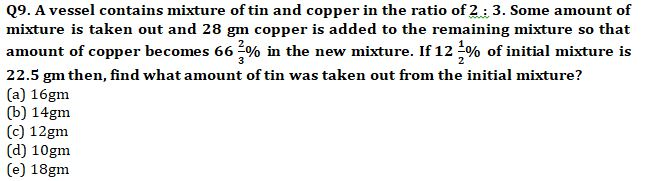Q10. Retailer mark up an article 35% above its cost price and earn Rs 96 by giving 20% discount on the marked price. If he sells article at 15% discount on marked price then, find retailer’s profit on selling one article.
(a) 118
(b) 177
(c) 236
(d) 214
(e) 154

Q11. Sum of age of A & B is 12 years more than sum of age of B, C & D. Average age of C & D is 29 yrs. Find average age of A & D if D is 12 years elder than C.
(a) 52.5 yrs
(b) 47.5 yrs
(c) 46.5 yrs
(d) 55.5 yrs
(e) 64 yrs

Q12. Mohit borrowed Rs. X on C.I. at the rate of 20% for three years. He paid at the end of first year 1/8th of amount and at the end of second year 1/6th of amount, if Mohit paid Rs. 15120 at the end of third year to complete his debt, then find the amount borrowed by Mohit?
(a) 24000 Rs.
(b) 16000 Rs.
(c) 12000 Rs.
(d) 36000 Rs.
(e) 42000 Rs.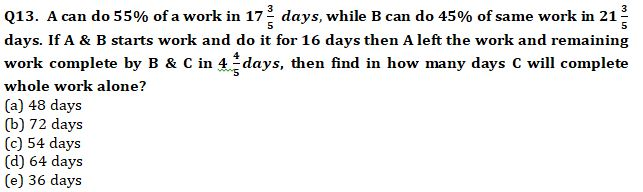Q14. 8 kg of type A wheat, at the rate of Rs. 60 per kg mixed with 12 kg of type B wheat, at the rate of Rs. X per kg. If the price of resulting mixture is Rs. 67.2 per kg, then find at what price should per kg type B wheat sold to make a profit of 25%?
(a) 84 Rs.
(b) 96 Rs.
(c) 104 Rs.
(d) 90 Rs.
(e) 78 Rs.

Q15. A bag contains 6 Red, 4 blue and 8 white ball, if three balls are drawn at random, find probability that one is Red and two are blue ?
(a) 3/68
(b) 5/68
(c) 7/68
(d) 9/68
(e) None of these

Practice More Questions of Quantitative Aptitude for Competitive Exams:

###### Study Plan for IBPS RRB PO/Clerk Prelims 2021

Solutions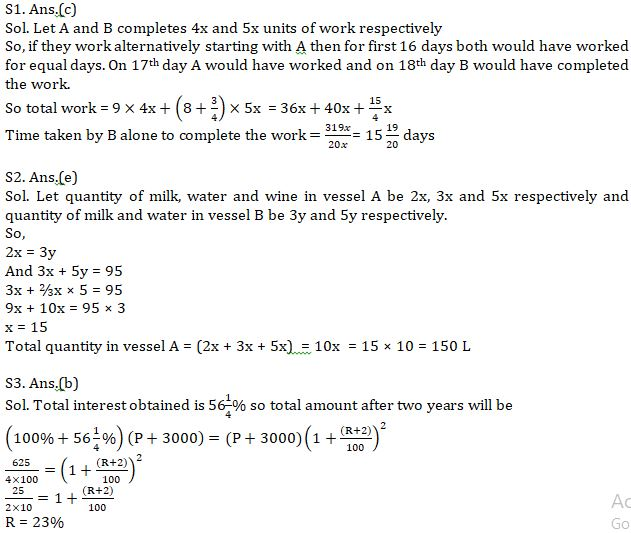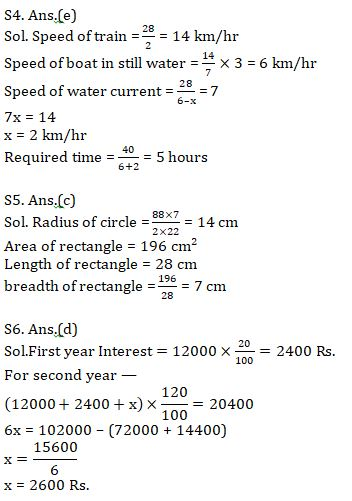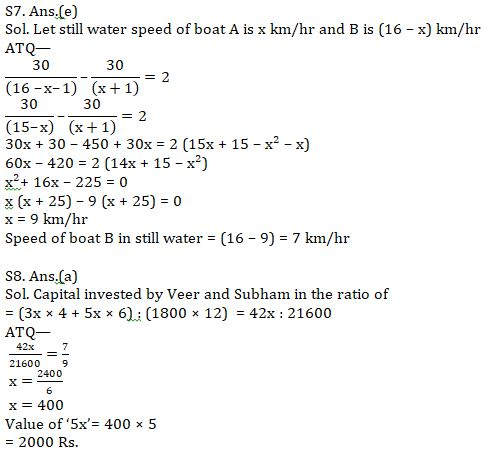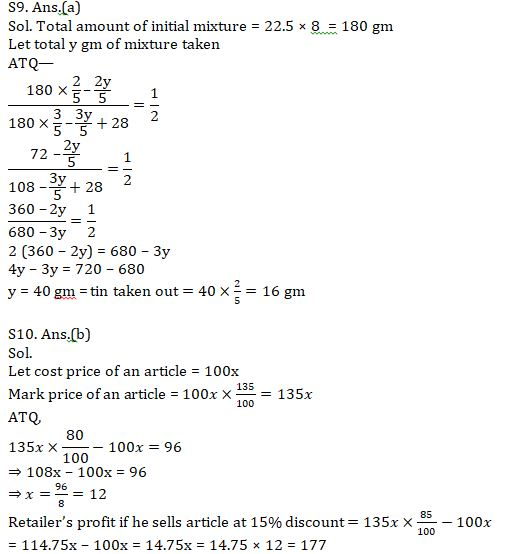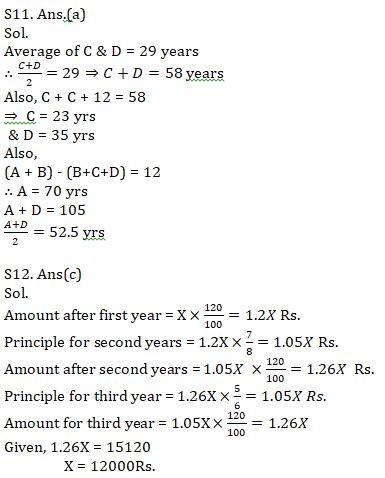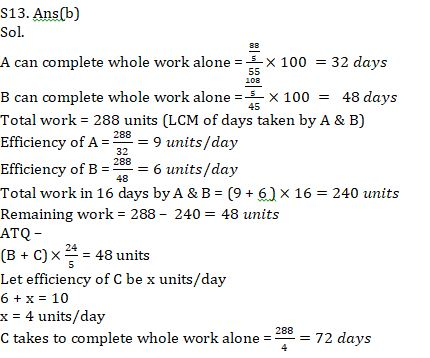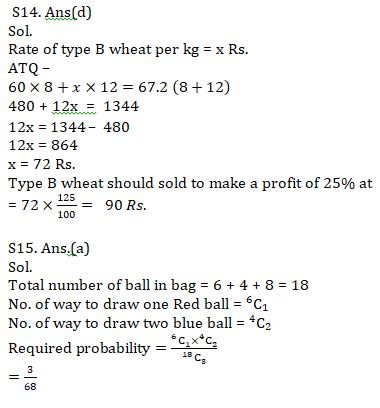#### Congratulations!Incorrect details? Fill the form again here

•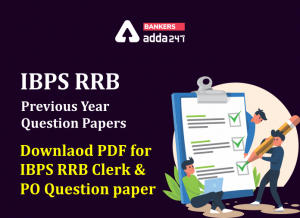IBPS RRB Previous Year Question Paper PD...
•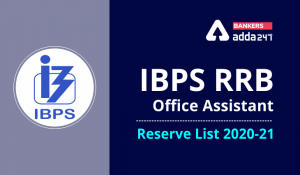IBPS RRB Clerk 2nd Reserve List 2022 Out...
•Quantitative Aptitude Quiz For IBPS RRB ...
•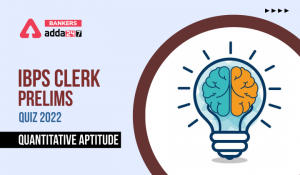Quantitative Aptitude Quiz For IBPS Cler...
•Reasoning Ability Quiz For IBPS RRB PO P...
•Quantitative Aptitude Quiz For IBPS RRB ...